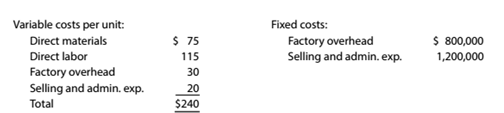Chapter 12, Problem 12.15E

Chapter
Section
Textbook Problem

Total cost concept of product costing Willis Products Inc. uses the total cost concept of applying the cost-plus approach to product pricing. The costs of producing and selling 200,000 units of medical tablets are as follows:Willis Products desires a profit equal to a 20% rate of return on invested assets of \$12,000,000.a.Determine the amount of desired profit from the production and sale of 200.000 units. b.Determine the total costs and the costamount per unitfor the production and sale of 200,000 units. c.Determine the total cost markup percentage per unit. d.Determine the selling price per unit.

To determine

Concept Introduction:

Cost Volume Profit (CVP) Analysis:

The Cost Volume Profit analysis is the analysis of the relation between cost, volume, and profit of a product. It analyzes the cost and profits at the different level of production, in order to determine the breakeven point and required the level of sales to earn the desired profit.

Contribution margin means the margin that is left with the company after recovering variable cost out of revenue earned by selling smart phones.

The formula for contribution margin is as follows:

Contribution margin = Sales - Variable cost.

Similarly contribution margin ratio = Contribution/sales

To Calculate:

The Cost amount per unit, the markup %, and the selling price per unit using the Variable cost

Explanation

The Cost amount per unit, the markup %, and the selling price per unit are calculated as follows:

 Variable Cost per unit (A) \$ 50.00 Desired Profit per unit (B) (240000/20000) \$ 12...

Still sussing out bartleby?

Check out a sample textbook solution.

See a sample solution

The Solution to Your Study Problems

Bartleby provides explanations to thousands of textbook problems written by our experts, many with advanced degrees!

Get Started

LO1 The purpose of depreciation is to record the assets market value in the accounting records.

College Accounting, Chapters 1-27 (New in Accounting from Heintz and Parry)

Describe the two-stage process associated with plantwide overhead rates.

Managerial Accounting: The Cornerstone of Business Decision-Making

What is structured systems design?

Pkg Acc Infor Systems MS VISIO CD

CAPM, PORTFOLIO RISK. AND RETURN Consider the following information for Stocks X, Y, and Z. The returns on the ...

Fundamentals of Financial Management, Concise Edition (with Thomson ONE - Business School Edition, 1 term (6 months) Printed Access Card) (MindTap Course List)